Experimental study of the dependences of retrieval efficiencies on time delay between magneto-optical-trap being turned off and optical storage
Chen Li-Rong, Xu Zhong-Xiao, Li Ping, Wen Ya-Fei, Zeng Wei-Qing, Wu Yue-Long, Tian Long, Li Shu-Jing, Wang Hai†,The State Key of Quantum Optics and Quantum Optics Devices, Institute of Opto-Electronics, Shanxi University, Taiyuan 030006, China

† Corresponding author. E-mail: wanghai@sxu.edu.cn

Project supported by the National Basic Research Program of China (Grant No. 2010CB923103) and the National Natural Science Foundation of China (Grant Nos. 11475109, 11274211, and 60821004).

Abstract
Abstract

We report an experimental study of electromagnetically induced transparency (EIT)-based light storage in a cloud of cold atoms loaded into a magneto-optical-trap (MOT). After the MOT is turned off, the retrieval efficiencies of right- and left-circularly polarized signal light fields each as a function of storage time are measured for different time delays between MOT off and the storage event, respectively. The results show that in the delay ranging from 0.015 ms to 3.5 ms, the retrieval efficiency for a zero-storage time (0.2 μs) and the storage lifetime can exceed 15% and 1.4 ms, respectively. The measured results will provide important help for optimizing the storage of the polarized entanglement photons in cold atomic ensembles.

1. Introduction

An elementary task in a quantum repeater is to build entanglement between two nodes. Such a task may be realized by mapping entangled two-photonic qubits in two atomic ensembles, respectively, and storing them for a desired time interval. The dynamic electromagnetically induced transparency (EIT) process in an atomic ensemble allows one to coherently transfer the quantum state between photons and spin waves, and has been developed into an effective optical quantum storage method.[12,13] Using an EIT-based storage scheme, Choi et al. have established entanglement between two quantum memories stored in cold atomic ensembles.

The entangled state between two photonic qubits A and B can be expressed by ψ = c1 |1〉A |0〉B + c2 |0〉A |1〉B (c1,2 ≠ 0), where |1〉 (|0〉) denotes the quantum state of a single photon pulse, for example, it may be the state of horizontal (vertical) polarization or presence (absence) of a single photon. Using spontaneous Raman scattering (SRS) in an atomic ensemble[5,1418] or spontaneous parametric down conversion (SPDC) in an optical cavity, one may generate photonic entanglement states capable of being stored. However, in order to suppress the generation of multi-photons, the probability for preparing the entangled states p should be very low, i.e., p ≪ 1. So, the generation trials of photonic entanglement states should be repeated many times for achieving a successful event. To realize storage of the entangled photonic qubits, the storage trials should be synchronized with the generation trials and also repeated many times until a successful generation event is heralded. The heralding of the successful generation event requires that the photonic entanglement states should be generated in a heralded[5,13] or an event-ready manner. On the other hand, the optical storage is imperfect in the usual case, which means that the retrieval efficiency η is less than 1. So, the required average trial number N for achieving a successful quantum memory event should be proportional to 1/. In the case where the storage medium is a cloud of cold atoms, one needs to first load atoms into MOT and then start a time sequence for operating the storage trials. Loading atoms requires an MOT-on period τM. Meanwhile the time sequence needs an interval τs, in which N = τs/T storage trials can be operated, where T is the period of each storage trial. So, one experimental circle should include loading and storing processes, which requires a total period of τc = τM + τs, corresponding to a repetition rate F = 1/τc. If the photons are produced and stored at j-th (1 ≤ j < N) trial, the time delay between MOT off and the storage will be τ = τ0 + (j −1)T (ττs, j = 1,2,…,n), where τ0 is the waiting time before the storage sequence starts. Since the number (temperature) of the trapped atoms will reduce (go up) with the increase of time delay τ, the retrieval efficiency and lifetime of the optical storage will decrease with the increase of delay τ. The required average trial number for achieving a successful storage event of the entangled photonic qubits is proportional to 1/η, which mainly depends on the retrieval efficiency. With the increase of delay τ, the retrieval efficiency will become very low, so we have to stop the storage trials and switch MOT on to start the next atomic loading. For achieving an optimal storage, one should understand the dependences of the retrieval efficiency on delay τ and then choose an appropriate limit of delay τ, i.e., interval τs. In recent years, retrieval efficiencies each as a function of storage time have been measured in EIT-based storage of classical light pulses in cold ensembles. Also, quantum memories for single photons have been experimentally implemented. However, the dependences of the retrieval efficiency on the delay between MOT off and the storage event have not been reported.

In this paper, we report an experimental study on EIT-based light storage in a cold atomic ensemble. The retrieval efficiencies of right- and left-circularly polarized signal light fields, each as the function of the storage time, are measured for different values of time delay τ, respectively. The results show that in the delay ranging from 0.015 ms to 3.5 ms, the storage lifetime and the retrieval efficiency for a zero-storage time (0.2 μs) can exceed 1.4 ms and 15%, respectively. Also, the dependence of the retrieval efficiency for a zero-storage time on the repetition rate F is measured. The result shows that the retrieval efficiency decreases with the increase of repetition rate F when the storage sequence period is a constant. The measured results will provide important help for optimizing the storage of the polarized entanglement photons in cold atomic ensembles.

2. Experimental setup

The involved levels of 87Rb atoms and the experimental setup are shown in Figs. 1(a)1(c), respectively, where |a〉 = |52S1/2,F = 1〉, |b〉 = |52S1/2,F = 2〉, and |e〉 = |52P1/2,F′ = 1〉. The right- and left-circularly polarized signal light fields ε±(z,t) are tuned to the transition |a〉 ↔ |e〉, respectively. The horizontally polarized controlling light field is composed of right- and left-circularly polarized components, which is tuned to the transition |b〉 ↔ |e〉. The atomic ensemble used for optical storage is a cigar-shaped cloud of cold 87Rb atoms which are provided by a two-dimensional (2D) magneto-optical trap (MOT). A pair of rectangular anti-Helmholtz coils with a size of 170 mm×65 mm is used to produce a magnetic field for the 2D MOT. The transverse gradient of the magnetic field is 15 Gs/cm (1 Gs = 10–4 T). The six trapping light beams have Gaussian profiles, whose diameters are all about 25 mm. The power of each trapping beam is about 20 mW. The size of the cold atomic cloud is about 4 mm×4 mm×7.5 mm.

 Figure Option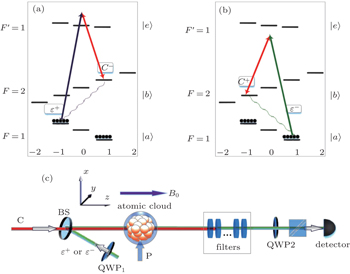Fig. 1. Overview of the experiment. Panels (a) and (b) show the three-level Λ-type EIT systems for the storage of the signal light field, ε+ and ε− denote right-circularly and left-circularly polarized signal light fields in a moderate magnetic field (B0 = 12 Gs), respectively. The unit 1 Gs = 10–4 T. C+ and C− denote right-circularly and left-circularly components of the linearly polarized controlling light field, respectively. Panel (c) shows the experimental setup, where P: pump laser beam; BS: polarization-insensitive beam splitter; QWP1,2: quarter-wave plates; C: linearly polarized controlling light (writing/reading) beam; OF: optical filters; PBS: polarization beam splitter.

The experimental setup is shown in Fig. 1(c). The signal and controlling light beams collinearly go through the cold atom cloud along the z direction, which can effectively suppress decoherence due to atomic motions. Their powers (diameters) are ∼65 μW (1 mm) and ∼ 12 mW (4 mm), respectively. Owing to the optical pumping by the stronger controlling laser, the most atoms are prepared into the state |a〉. For further preparing the atoms into Zeeman sublevels |am=−1〉 or |am=1〉 with equal population (m is the magnetic quantum number), we use a π-polarized pumping laser beam P, which drives the transition |5S1/2,F = 1,m = 0〉 ↔ |52P3/2F′ = 0,m = 0〉 and propagates through the atoms along the x direction. Its power and diameter are ∼ 4 mW and ∼ 20 mm, respectively. The dephasing rate of the magnetic-field-sensitive (MFS) coherence is large, which is one of main limits to the storage lifetime. In the present work, we use the scheme demonstrated in our previously published paper to overcome this limit. As shown in Figs. 1(a) and 1(b), we use a moderate magnetic field (12 Gs) to lift the Zeeman degeneracy of the states |a〉 and |b〉, and then form two three-level Λ-type EIT systems, one is |am=−1〉 − |em=0〉 − |bm=1〉, which is coupled by the right-circularly polarized signal light fields ε+ and left-circularly polarized controlling component C, and the other is |am=1〉 − |em=0〉 − |bm=−1〉, which is coupled by the left-circularly polarized signal field ε and right-circularly polarized component of controlling field C+. The mapping between the signal light fields ε±(z,t) and the spin waves may be described by the two dark-state polaritons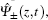[9,32]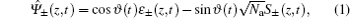respectively, where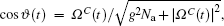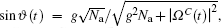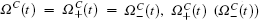is the Rabi frequency of the right-(left-) circularly polarized controlling components C±, Na is the total number of atoms, g is the atom-field coupling constant, S±(z,t) are the spin waves associated with the magnetic-field-insensitive (MFI) coherences |am=−1〉 ↔ |bm=1〉 and |am=1〉 ↔ |bm=−1〉, respectively, and are expressed as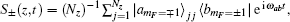with ωab being the frequency of the transition |a〉 ↔ |b〉, and Nz(≫ 1) being the number of atoms in an interval Δz. By adiabatically switching off the controlling light beam, cos ϑ(t) will be rotated from 0 to π/2 and the input pulse of signal field ε+(z,t) or ε(z,t) will be transferred into the MFI spin wave S+(z,t) or S(z,t),respectively. However, if the controlling light beam is adiabatically switched on, cos ϑ(t) will be rotated from π/2 to 0 and the pulses of signal field ε+(z,t) or ε(z,t) will be retrieved from the spin waves S+ and S, respectively. Such two processes correspond to the “writing” and “reading” processes respectively. Since the signal fields are only stored into the MFI spin waves, we can achieve a long-lived optical storage. The time-dependent retrieval efficiencies η±(t) for right- and left-circularly polarized signal light fields are defined as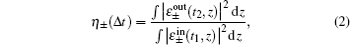respectively, where Δt = t2t1 is the storage time,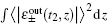corresponds to the retrieved photon numbers of right- and left-circularly polarized signal light fields at time t2, respectively, while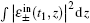corresponds to the input photon numbers of right- and left-circularly polarized signal light fields at the initial time t1, respectively.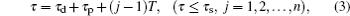which depends on the trial j. The period of the storage sequence in each circle can be written as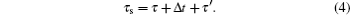The repetition rate F can be written as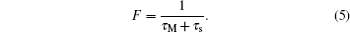From this relation, we can see that F decreases with the increase of MOT-on (MOT-loading) period τM when τs is kept constant.

 Figure Option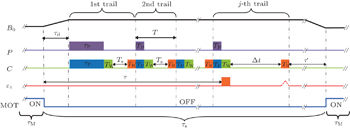Fig. 2. Time sequence of an experimental circle. ε± denotes the time sequence of signal light, which shows that the signal pulse is stored at the j-th storage trial. C, P, and B0 denote the time sequences of the controlling light, pumping light, and guiding magnetic field, respectively. τM is the MOT-on time interval; τd = 10 μs is the waiting time for MOT magnetic field decaying to zero. τP = 5 μs is the atomic preparation time in the first trial. TP = 100 ns is the time window of the atomic preparation in the j-th (j > 1) trial. TW = TR = 100 ns and TS = 200 ns are the writing, reading, and waiting windows, respectively.
3. Results and discussion

We first measure the dependences of the retrieval efficiencies η± for a zero-storage time (Δt = 0.2 μs) on time delay τ, respectively. In the measurements, we keep τM = 91.5 ms and τs = 8.5 ms, so the repetition rate is calculated to be F = 10 Hz. The red-circle and black-square curves in Fig. 3 are the measured retrieval efficiencies for the right- and left-circularly polarized signal light fields each as a function of delay time τ, respectively. The measured results for right- and left-circularly polarized signal light fields are very close to each other and decrease with the increase of time delay τ. Within the delay range from 0.015 ms to 3.5 ms, the retrieval efficiency may exceed 15%. For understanding the decreases of the retrieval efficiencies with the increase of delay τ, we measure the optical depths of the right- and left-circularly polarized signal light fields as a function of the delay τ, respectively. The measured results are shown in Fig. 4, which decrease with the increase of delay τ; the reason for this is that the number of atoms drifting out of the signal–beam region increases with the increase of τ. Considering the dependences of the retrieval efficiencies on the optical depth,[26,33] we may understand the results in Fig. 3.

 Figure Option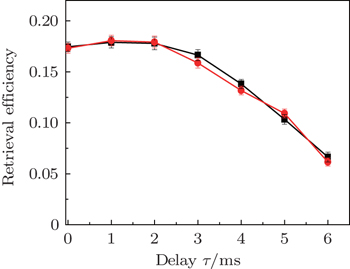Fig. 3. Retrieval efficiencies each as a function of the time delay τ. Red circle and black square dots are the measured results for the right- and left-circularly polarized signal light fields, respectively.
 Figure Option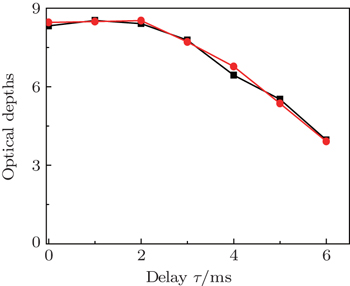Fig. 4. Optical depths each as a function of the time delay τ. Red circle and black square dots are the measured results for the right- and left-circularly polarized signal light fields, respectively.

Next, we measure the dependences of retrieval efficiencies on the storage time Δt for several different values of delay τ. In the measurement, F is still kept at 10 Hz. The red circle and black square dots in Fig. 5(a)5(f) are the measured retrieval efficiencies of right- and left-circularly polarized signal light fields for delays of 0.015 ms, 0.1 ms, 1.5 ms, 3.5 ms, 5 ms, and 6 ms, respectively. The solid lines in Figs. 5(a)5(f) are the corresponding fittings to the measured data using ηt) = η0 e–Δt/τ. According to these fittings, we may obtain 1/e lifetimes for the different delays and then plot the dependence of the lifetime on the delay τ, which is shown in Fig. 6. From this figure, we can see that the maximum lifetime is about 1.7 ms, which occurs in the delay range from 0.1 ms to 1.5 ms. When the delay is beyond the range, the storage lifetime will reduce. The reason may be explained as follows. If the delay is very short (τ = 0.015 ms), the MOT magnetic field will not decay to zero within such a short delay. In this case, the remaining magnetic field will cause significant inhomogeneous Zeeman broadening of the spin waves, which will lead to a reduction of the storage lifetime. On the other hand, if the delay τ is very long, the temperature of the cold atoms will acquire a significant increase, which leads to the decrease of storage lifetime with the delay.

 Figure Option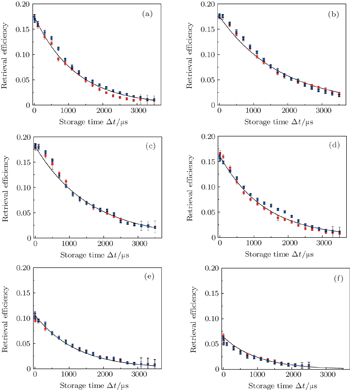Fig. 5. Variations of retrieval efficiency with storage time Δt for the time delays of 15 μs (a), 100 μs (b), 1500 μs (c), 3500 μs (d), 5000 μs (e), and 6000 μs (f), respectively. In panels (a)–(f), red circle and black square dots are the measured results for the right- and left-circularly polarized signal light fields, respectively, and solid lines are the fittings based on η(Δt) = η0e–Δt/τ.
 Figure Option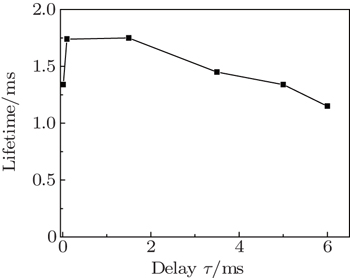Fig. 6. Lifetime as a function of time delay τ.

We then measure the dependences of retrieval efficiencies on repetition rate F. In the measurement, the period of storage sequence is kept at τs = 8.5 ms and the repetition rate F is changed by varying the MOT-on period τM. The storage of the right- or left-circularly polarized signal light fields are performed in the first trial, and their retrieval efficiencies are measured for the storage time Δt = 0.2 μs, respectively. The red circle and black square dots in Fig. 7 are the measured results, which show that the retrieval efficiencies decrease with the increase of F. These results can be easily explained as follows. The number of trapped atoms can be written as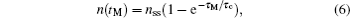where τc is the lifetime of the trap, nssd2 is steady-state number of trapped atoms, d is the diameter of the cooling laser beam. Since the optical depth (OD) is proportional to the number of trapped atoms n(τM), it can be written as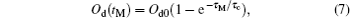where Od0nssd2. From this relation, we can see that OD decreases with the decrease of MOT-on period τM, which makes the retrieval efficiencies decrease with the increase of repetition rate F. If one hopes to improve the retrieval efficiencies in the case of high F, they need to increase OD by expanding the diameters of the trapping laser beams.

 Figure Option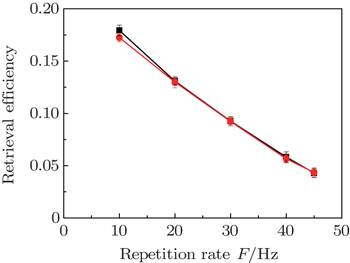Fig. 7. Measured variations of retrieval efficiencies with repetition rate. Red circle and black square dots are the measured results for the right- and left-circularly polarized signal light fields, respectively.
4. Conclusions

We study the optical storage of right- and left-circularly polarized signal light fields in a cloud of cold atoms trapped in a MOT. The retrieval efficiencies of right- and left-circularly polarized signal light fields each as a function of storage time are measured for different time delays between MOT off and the storage event, respectively. The measured results show that the retrieval efficiencies decrease with the increase of delay τ. In the delay times ranging from 0.015 ms to 3.5 ms, the storage lifetime and the retrieval efficiency for a zero-storage time (0.2 μs) can exceed 1.4 ms and 15%, respectively. The measured retrieval efficiencies of right- and left-circularly polarized signal light fields are close to each other, the present storage system can be used to store polarized photonic qubits. The dependence of the retrieval efficiency for the zero-storage time (0.2 μs) on repetition rate F is also measured. The further improvement of the retrieval efficiencies in the case of high repetition rate F requires one to expand the diameters of the trapping laser beams. The present results will provide important help for optimizing the storage of the polarized entanglement photons.

Reference
 1 Duan L MLukin MCirac J IZoller P 2001 Nature 414 413 2 Zhao BChen Z BChen Y ASchmiedmayer JPan J W 2007 Phys. Rev. Lett. 98 240502 3 Simon Cde Riedmatten HAfzelius MSangouard NZbinden HGisin N 2007 Phys. Rev. Lett. 98 190503 4 Chen Z BZhao BChen Y ASchmiedmayer JPan J W 2007 Phys. Rev. A 76 022329 5 Choi K SDeng HLaurat JKimble H J 2008 Nature 452 67 6 Bai J HLu X GMiu X XPei L YWang MGao Y LWang R QWu L AFu P MZuo Z C 2015 Acta Phys. Sin. 64 034206 (in Chinese) 7 Bian C LZhu JLu J WYan J LChen L QWang Z BOu Z YZhang W P 2013 Acta Phys. Sin. 62 174207 (in Chinese) 8 Wang BLi S JChang HWu H BXie C DWang H2005Acta Phys. Sin.544136(in Chinese) 9 Fleischhauer MLukin M D 2000 Phys. Rev. Lett. 84 5094 10 Liu CDutton ZBohroozi C HHau L V 2001 Nature 409 490 11 Phillips D FFleischhauer AMair AWalsworth R LLukin M D 2001 Phys. Rev. Lett. 86 783 12 Lvovsky A ISanders B CTittel W 2009 Nat. Photon. 3 706 13 Sangouard NSimon Cde Riedmatten HGisin N 2011 Rev. Mod. Phys. 83 33 14 Matsukevich D NKuzmich A 2004 Science 306 663 15 Chen SChen Y AZhao BYuan Z SSchmiedmayer JPan J W 2007 Phys. Rev. Lett. 99 180505 16 Zhang WDing D SPan J SShi B S 2014 Chin. Phys. Lett. 31 064208 17 Lu X SChen Q FShi B SGuo G C 2009 Chin. Phys. Lett. 26 064204 18 Liu RYu Y FZhang Z M 2014 Acta Phys. Sin. 63 144203 (in Chinese) 19 Bao X HQian YYang JZhang HChen Z BYang TPan J W 2008 Phys. Rev. Lett. 101 190501 20 Sangouard NSimon CZhao BChen Y Ade Riedmatten HPan J WGisin N 2008 Phys. Rev. A 77 062301 21 Han Y XLiu Y HZhang C HLi S JWang H 2009 Chin. Phys. Lett. 26 023201 22 Ji Z HYuan J PZhao Y TChang X FXiao L TJia S T 2014 Chin. Phys. B 23 113702 23 Yan S BGeng TZhang T CWang J M 2006 Chin. Phys. 15 1746 24 Riedl SLettner MVo CBaur SRempe GDürr S 2012 Phys. Rev. A 85 022318 25 Xu Z XWu Y LTian LChen L RZhang Z YYan Z HLi S JWang HXie C DPeng K C 2013 Phys. Rev. Lett. 111 240503 26 Chen Y HLee M JWang I CDu SChen Y FChen Y CYu I 2013 Phys. Rev. Lett. 110 083601 27 Lettner MMücke MRiedl SVo CHahn CBaur SBochmann JRitter SDürr SRempe G 2011 Phys. Rev. Lett. 106 210503 28 Chaneliere TMatsukevich D NJenkins S DLan S YKennedy T A BKuzmich A 2005 Nature 438 833 29 Zhang HJin X MYang JDai H NYang S JZhao T MRui JHe YJiang XYang FPan G SYuan Z SDeng YChen Z BBao X HChen SZhao BPan J W 2011 Nat. Photon. 5 628 30 Zhao BChen Y ABao X HStrassel TChuu C SJin X MSchmiedmayer JYuan Z SChen SPan J W 2008 Nat. Phys. 5 95 31 Dudin YJenkins SZhao RMatsukevich DKuzmich AKennedy T 2009 Phys. Rev. Lett. 103 020505 32 Xu Z XWu Y LLiu H LLi S JWang H 2013 Phys. Rev. A 88 013423 33 Zhang S CZhou S YLoy M MWong G KDu S W 2011 Opt. Lett. 36 4530 34 Gibble K EKasapi SChu S 1992 Opt. Lett. 17 526